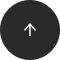Standard SQL

# How to Order Rows by Group Sum in SQL

#### Operators:

ORDER BY, DESC, SUM(), GROUP BY

#### Problem:

You’d like to order rows by the sums generated by a group of records.

#### Example:

Our database has a table named `training` with data in four columns: id, login, year, and score.

1Andy201824
2Lucy201925
3Andy201920
4Lucy201816
5Gary201918
6Gary201819
7Gary201722
8Lucy201721
9Andy201726

Let’s get the login name of each player along with the total sum of score across all years, putting records in descending order according to players’ total scores.

#### Solution:

We’ll use the operator ORDER BY to order records based on the aggregate function `SUM()`, which calculates the total score for each player across all years.

Here’s the query you’d write:

```SELECT login,
SUM(score) AS total_score
FROM training
ORDER BY SUM(score) DESC;
```

Here’s the result:

Andy70
Lucy62
Gary59

#### Discussion:

Use ORDER BY if you want to order rows according to a value returned by an aggregate function like `SUM()`. The ORDER BY operator is followed by the aggregate function (in our example, `SUM()`). DESC is placed after this function to specify a descending sort order. Thus, the highest aggregate values are displayed first, then progressively lower values are displayed. To sort in ascending order, you can specify ASC or simply omit either keyword, as ascending is the default sort order.

In the query above, we select each player’s login and the sum of their score for all years. This total score is calculated using SUM() with the score column as an argument. We add an alias for this aggregate value (`SUM(score) AS total_score`); you can use this alias instead of the aggregate function in the ORDER BY clause (`ORDER BY total_score DESC`).

Notice that we include login in the GROUP BY. If we include a column in SELECT, we must also use the column in GROUP BY. In this example, we use GROUP BY clause followed by the column login because we put this column in the SELECT. Notice that GROUP BY is placed before ORDER BY in the query.

#### Recommended articles: